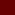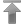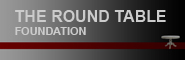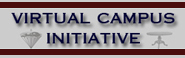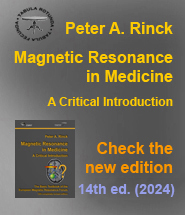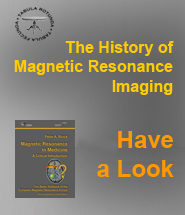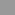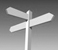Contenido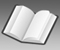Datos# Glosario

 a b c d e f g h i j k l m n o p q r s t u v w x y z

### L

LAO: left anterior oblique (projection through the human body).

Larmor equation: states that the frequency of precession of the nuclear magnetic mo­ment is proportional to the magnetic field.
ω0 = -γ × B0 (radians per second); or
ν0 = -γ × B0/2π (Hertz),
where ω0 and ν0, respectively, are the frequency, γ is the gyromagnetic ratio, and B0 is the magnetic induction field. The negative sign indicates the direction of rotation.

Larmor frequency (ω0 or ν0): the frequency at which magnetic resonance can be ex­ci­ted; given by the Larmor equation. By varying the magnetic field across the body with a gradient magnetic field, the corresponding variation of the Larmor frequency can be used to encode position. For protons (hydrogen nuclei), the Larmor frequency is 42.58 MHz/ Tesla.

Lattice: the magnetic and thermal environment with which nuclei exchange energy in longitudinal relaxation.

Line imaging → Sequential line imaging.

Line scanning → Sequential line imaging.

Line width: width of line in spectrum; related to the reciprocal of the transverse re­la­xa­tion time (T2* in practical systems). Measured in units of frequency, generally at the half- maximum points.

Localized spectroscopy: the acquisition of spectra from a region within a sample. The region is usually defined using selective RF pulses and field gradients.

Longitudinal magnetization (Mz): component of the macroscopic magnetization vec­tor along the static magnetic field. Following excitation by RF pulse, Mz will approach its equilibrium value M0, with a characteristic time constant T1.

Longitudinal relaxation: return of longitudinal magnetization to its equilibrium value after excitation; requires exchange of energy between the nuclear spins and the lattice.

Longitudinal relaxation time → T1.

Lorentzian line: usual shape of the lines in an NMR spectrum, characterized by a cen­tral peak with long tails; proportional to 1/[(1/T2)² + (ν-ν0)²], where ν is frequency and ν0 is the frequency of the peak (i.e. central resonance frequency).

Missing terms? Send us an e-mail. We'll add them ...